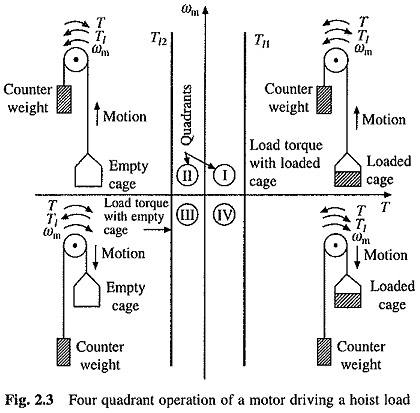As we know already, the nature of Classification of Load Torques depends on particular application. A low speed hoist is an example of a load where the torque is constant and independent of the speed (Fig. 2.3).At low speeds, windage torque is negligible. Therefore, net torque is mainly due to gravity which is constant and independent of speed. There are drives where coulomb friction dominates over other torque components. Consequently, torque is independent of speed, e.g. paper mill drive.Fans, compressors, aeroplanes, centrifugal pumps, ship-propellors, coilers, high speed hoists, traction etc. are example of the case where load torque is a function of speed. In fans, compressors and aeroplanes, the windage dominates, consequently, load torque is proportional to speed squared (Fig. 2.7(a)).

Windage is the opposition offered by air to the motion. Similar nature of Classification of Load Torques can be expected when the motion is opposed by any other fluid, e.g. by water in centrifugal pumps and ship-propellors, giving the same characteristic as shown in Fig. 2.7(a). In a high speed hoist, viscous friction and windage also have appreciable magnitude, in addition to gravity, thus giving the speed-torque curve of Fig. 2.7(b). Nature of speed-torque characteristic of a traction load when moving on a levelled ground is shown in Fig. 2.7(c). Because of its heavy mass, the stiction is large. Near zero speed, net torque is mainly due to stiction.

The stiction however disappears at a finite speed and then windage and viscous friction dominate. Because of large stiction and need for accelerating a heavy mass, the motor torque required for starting a train is much larger than what is required to run it at full speed. Torque in a coiler drive is also a function of speed. It is approximately hyperbolic in nature as shown in Fig. 2.7(d). The developed power is nearly constant at all speeds.

Figure 2.7(c) shows the traction load torque to be function of only speed, because we have assumed a levelled ground. In actual practice the train has to negotiate upward and downward slopes. Consequently, a torque due to gravity, which varies with position is also present. Furthermore, when a train takes a turn the frictional force on wheels changes substantially. Thus, traction is an example where the load torque also depends on position or path followed.